### 克莱斯勒(进口) 克莱斯勒300C(进口)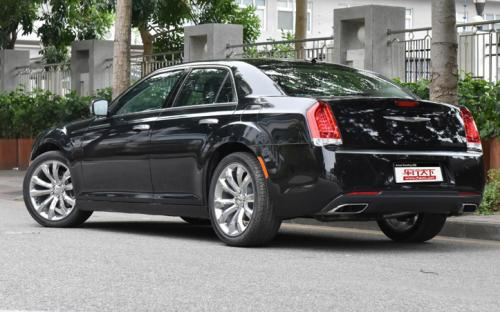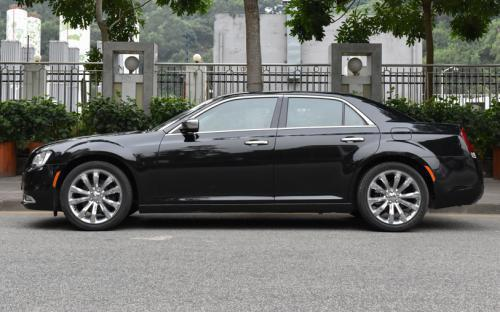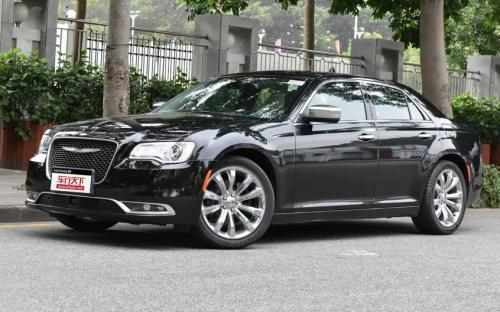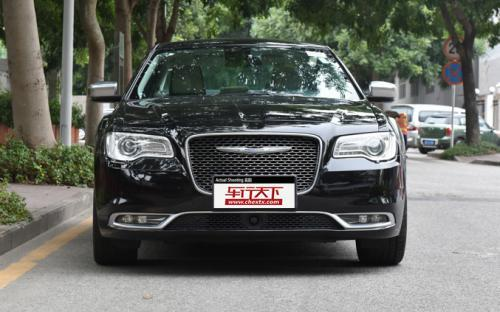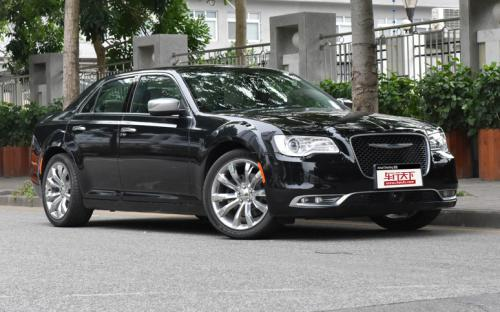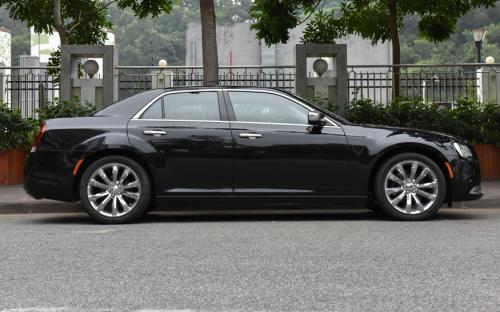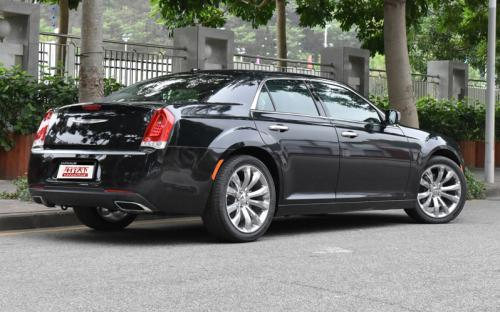9 种颜色可选2016款最低售价：39.99 万元起

5066(mm)1902(mm)1488(mm)##### 配置亮点：
• 胎压监测装置

• ISOFIX儿童座椅接口

• 车身稳定控制(ESC/ESP/DSC等)

• 电动天窗

• 定速巡航

• 后倒车雷达

• 真皮座椅

• GPS导航系统

• 氙气大灯

• 后视镜加热

• 提交
2016款 3.0L 超越版 (128张)
• 2016款 3.0L 超越版 (128张)
• 2016款 3.6L 尊崇版 (97张)
• 克莱斯勒(进口) 克莱斯勒300C(进口) 绕车实拍• 克莱斯勒(进口) 克莱斯勒300C(进口) 在售车型

排量 车型 厂商指导价 本地最低报价 购车工具
3.0L
3.0L 超越版 8挡手自一体
39.99万
39.99万

3.6L 尊崇版 8挡手自一体
43.19万
43.19万

3.6L
3.0L 超越版 8挡手自一体
39.99万
39.99万

3.6L 尊崇版 8挡手自一体
43.19万
43.19万

克莱斯勒(进口) 克莱斯勒300C(进口) 经销商

查看更多 >>

### 克莱斯勒(进口) 克莱斯勒300C(进口) 动力加速

克莱斯勒300C(进口) 0-100公里加速时间分布在 0.0-秒 属于 超跑级

动力级别 加速时间 车型

克莱斯勒(进口) 克莱斯勒300C(进口) 视频

克莱斯勒(进口) 克莱斯勒300C(进口) 新闻资讯

# 克莱斯勒新款300C正式上市 售价39.99万起

上市新车 超过8550次关注

2015年12月2日，克莱斯勒宣布新款300C正式上市，新车克莱斯勒新款300C针对一些细节进行了改进，水晶的网状进气格栅，而前保险杠、前大灯、雾灯造型也有所变化；提供...

# 非主流之选 50万内进口中型车推荐

导购 超过2435次关注

谈到克莱斯勒300C，我相信很多国内消费者都不会感到陌生。当年第十代车型国产后，凭借豪华且大气的外观曾经得到不少国内消费者的青睐，不过受到油耗过高以及后来厂...

# 克莱斯勒300C 3.0L性能测试

评测 超过2615次关注

这台克莱斯勒300C3.0L卓越版（以下简称300C）配备了一台3.0LPentastarVVTV6自然吸气发动机，最大功率234马力/6350rpm，峰值扭矩285牛·米/4400rpm，指导售价在40万...

# 克莱斯勒300S锋尚版实拍 加入运动元素

导购 超过3703次关注

谈及克莱斯勒300C，相信许多国内消费者和车友都不陌生，其凭借着豪华大气的外观赢得了不少喜欢美式豪放风格车迷的喜爱。前段时间，厂家为了进一步丰富300C的产品线...

# 克莱斯勒300C 3.0L于2014北京车展上市

新闻 超过2371次关注

在本届北京车展上，克莱斯勒300C3.0L车型正式上市，该车仅有一款，售价为39.99万元。

# 克莱斯勒300C 3.0L车型首发 预售40万元

新闻 超过2502次关注

克莱斯勒正式发布了300C3.0L车型，相比于在售的3.6L车型，克莱斯勒300C3.0L车型将会有更高的性价比。根据官方目前透露的消息，该车型将于今年4月的北京车展后正式上...

# 克莱斯勒召回进口300S车型 涉及151辆

新闻 超过2510次关注

日前，克莱斯勒（中国）汽车销售有限公司向国家质检总局备案了召回计划，将自2014年5月15日起，召回部分进口款克莱斯勒2013款/2014款300S车型。据该公司统计，在中...

猜你喜欢

﻿
• 快速找车
• 选择品牌
• 选择品牌
• A  奥迪
• A  阿斯顿·马丁
• A  阿尔法·罗密欧
• B  宝沃
• B  布加迪
• B  巴博斯
• B  保时捷
• B  宾利
• B  奔驰
• B  宝马
• B  本田
• B  别克
• B  标致
• B  比亚迪
• B  宝骏
• B  北汽制造
• B  北汽新能源
• B  北汽幻速
• B  北汽威旺
• B  北京汽车
• B  奔腾
• B  北汽绅宝
• C  长安
• C  长安商用
• C  长城
• C  昌河
• D  大众
• D  道奇
• D  DS
• D  东南
• D  东风风神
• D  东风风行
• D  东风小康
• D  东风风度
• D  东风
• F  福特
• F  丰田
• F  菲亚特
• F  法拉利
• F  福田
• F  福迪
• F  福汽启腾
• G  观致
• G  广汽传祺
• G  广汽吉奥
• G  GMC
• H  红旗
• H  汉腾汽车
• H  哈弗
• H  哈飞
• H  海格
• H  海马
• H  华颂
• H  黄海
• H  华泰
• H  恒天
• J  吉利汽车
• J  捷豹
• J  Jeep
• J  江淮
• J  江铃
• J  金杯
• J  九龙
• J  金旅
• K  凯翼
• K  凯迪拉克
• K  克莱斯勒
• K  科尼塞克
• K  卡威
• K  开瑞
• L  路虎
• L  林肯
• L  劳斯莱斯
• L  兰博基尼
• L  雷克萨斯
• L  铃木
• L  雷诺
• L  理念
• L  力帆
• L  莲花汽车
• L  猎豹
• L  路特斯
• L  陆风
• M  马自达
• M  MG
• M  MINI
• M  玛莎拉蒂
• M  摩根
• M  迈凯轮
• N  纳智捷
• O  欧宝
• O  讴歌
• O  欧朗
• Q  奇瑞
• Q  起亚
• Q  启辰
• R  日产
• R  荣威
• R  瑞麒
• S  三菱
• S  斯威汽车
• S  萨博
• S  smart
• S  斯柯达
• S  斯巴鲁
• S  思铭
• S  双龙
• S  上汽大通
• S  双环
• T  特斯拉
• T  腾势
• W  沃尔沃
• W  五菱汽车
• W  五十铃
• W  威兹曼
• W  威麟
• X  现代
• X  雪佛兰
• X  雪铁龙
• X  西雅特
• Y  一汽
• Y  英菲尼迪
• Y  英致
• Y  依维柯
• Y  野马汽车
• Y  永源
• Z  众泰
• Z  中华
• Z  中兴
• Z  知豆
• 选择车系
• 选择车系
• 车型对比
• 选择品牌
• 选择品牌
• A  奥迪
• A  阿斯顿·马丁
• A  阿尔法·罗密欧
• B  宝沃
• B  布加迪
• B  巴博斯
• B  保时捷
• B  宾利
• B  奔驰
• B  宝马
• B  本田
• B  别克
• B  标致
• B  比亚迪
• B  宝骏
• B  北汽制造
• B  北汽新能源
• B  北汽幻速
• B  北汽威旺
• B  北京汽车
• B  奔腾
• B  北汽绅宝
• C  长安
• C  长安商用
• C  长城
• C  昌河
• D  大众
• D  道奇
• D  DS
• D  东南
• D  东风风神
• D  东风风行
• D  东风小康
• D  东风风度
• D  东风
• F  福特
• F  丰田
• F  菲亚特
• F  法拉利
• F  福田
• F  福迪
• F  福汽启腾
• G  观致
• G  广汽传祺
• G  广汽吉奥
• G  GMC
• H  红旗
• H  汉腾汽车
• H  哈弗
• H  哈飞
• H  海格
• H  海马
• H  华颂
• H  黄海
• H  华泰
• H  恒天
• J  吉利汽车
• J  捷豹
• J  Jeep
• J  江淮
• J  江铃
• J  金杯
• J  九龙
• J  金旅
• K  凯翼
• K  凯迪拉克
• K  克莱斯勒
• K  科尼塞克
• K  卡威
• K  开瑞
• L  路虎
• L  林肯
• L  劳斯莱斯
• L  兰博基尼
• L  雷克萨斯
• L  铃木
• L  雷诺
• L  理念
• L  力帆
• L  莲花汽车
• L  猎豹
• L  路特斯
• L  陆风
• M  马自达
• M  MG
• M  MINI
• M  玛莎拉蒂
• M  摩根
• M  迈凯轮
• N  纳智捷
• O  欧宝
• O  讴歌
• O  欧朗
• Q  奇瑞
• Q  起亚
• Q  启辰
• R  日产
• R  荣威
• R  瑞麒
• S  三菱
• S  斯威汽车
• S  萨博
• S  smart
• S  斯柯达
• S  斯巴鲁
• S  思铭
• S  双龙
• S  上汽大通
• S  双环
• T  特斯拉
• T  腾势
• W  沃尔沃
• W  五菱汽车
• W  五十铃
• W  威兹曼
• W  威麟
• X  现代
• X  雪佛兰
• X  雪铁龙
• X  西雅特
• Y  一汽
• Y  英菲尼迪
• Y  英致
• Y  依维柯
• Y  野马汽车
• Y  永源
• Z  众泰
• Z  中华
• Z  中兴
• Z  知豆
• 选择车系
• 选择车系
• 选择车型
• 选择车型
• 意见反馈